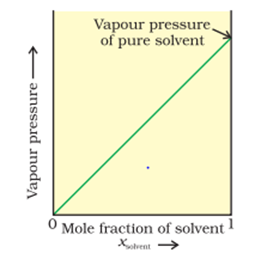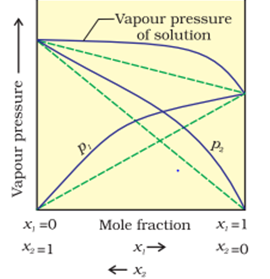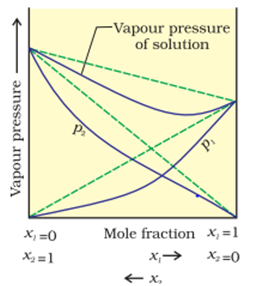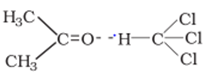# Ideal and Non- Ideal Solutions

## Ideal and Non- Ideal Solutions

Liquid-liquid solutions can be classified into ideal and non-ideal solutions on the basis of Raoult’s law

### Ideal solution

An ideal solution is a solution which always obeys Raoult’s law under all conditions of temperature and pressure.
Characteristics of ideal solution
1.Should obey Raoult’s law
i. e. $P_A = P_A^O \chi _A$ & $P_B = P_B^O \chi _B$
2. $\Delta H_{mix}=0$
3. $\Delta V_{mix}=0$

Characteristic Curve:When a graph is plotted between V. P. & M. F. then a straight line is obtained for ideal solution

Condition:
Ideal solution is obtained when the intermolecular force existing between the particles of A, existing between the particles of B & existing between the particles of A & B are nearly the same.
Example
• Methanol + Ethanol
• Benzene + Toulene
• Heptane + Octane

Formula
When both A and B are volatile
$P_A = P_A^O \chi _A$
$P_B = P_B^O \chi _B$
$P_S = P_A + P_B$
So,
$P_S = P_A^O \chi _A + P_B^O \chi _B$
When A is volatile and B is non – volatile
$P_A = P_A^O \chi _A$
$P_B = 0$
$P_S = P_A + P_B$
$P_S = P_A^O \chi _A$

### Non -Ideal solution

Non - ideal solutions are those solutions which do not always obey Roault’s law.
Non - ideal solution are of two types:-
 Non - ideal solution showing ‘+’ Deviation from Roault’s law Non - ideal solution showing ‘-‘ Deviation on from Raoult’s law These are the solution in which the partial vapour pressure of a component ($P_A$) is more than the expected value ($P_A^O \chi _A$) Characteristic: (i) $\Delta H_{mix} > 0$ Such solution are endothermic i. e. they absorb heat from the surrounding & therefore they appear to be cool when mixed. (ii) $\Delta V_{mix} > 0$Condition: It is observed when the force of attraction between A & B particles is less than the fore of attraction.existing between the particles of A and the particles of B. Eg: Ethanol + Cycbhexane In order to mix cyclohexane & Ethanol the hydrogen bonding in Ethanol needs to be broken. Breaking of hydrogen bonds require energy which is obtained from the surrounding. Thus, the mixture appears to be cool when mixed. The intermolecular force existing between Ethanol & Cyvbhexane is weaker than the hydrogen bonding in Ethanol. Due to decrease in the inter molecular force the inter particle distance increases & the volume is increased. Thus $\Delta V_{mix} > 0$ with increase in temperature the solubility increases. The partial vapour pressure of a component ($P_A$) is less than the expected value ($P_A^O \chi _A$) Characteristic: (i) $\Delta H_{mix} < 0$ Such solutions are endothermic and they lose heat to the surrounding thus they become hot when mixed. (ii) $\Delta V_{mix} < 0$Condition: It is observed when the inter molecular force existing between the particles of A & B is greater than the force of attraction existing the particles of A or the particles of B. Eg: Chloroform + acetoneWhen chloroform & acetone mixed then hydrogen is formed between them to formation of hydrogen bonding energy is lost to the surrounding the mixture appears hot. The intermolecular force existing between chloroform acetone is greater the intermolecular force exist in either chloroform molecules or acetone molecule. Due increases in the intermolecular force the interparticle –e decreases and hence volume also decreases. Thus $\Delta V_{mix} < 0$ with increase in temperature the solubility decreases

## ZEOTROPIC MIXTURE OR CONSTANT BOILING MIXTURE

• These are the mixtures which have same composition both in liquid state as well as in vapour state i.e. xA = yA & xB = yB
• These are the mixtures whose components boil at a fixed temperature. Thus, the components of Azeotropic mixture cannot be separated by the fractional distillation.
Types of Azeotropic mixture:-
 Minimum Boiling Azeotropic Maximum Boiling Azeotropic Those soln which show +ve deviation from Raoult’s law form minimum boiling Azeotrope   Eg: 95% Ethanol + 5% water Those soln which show -ve deviation from Roault’s law from maximum boiling Azeotropes. Eg: 68% HNO3 + 32% water.